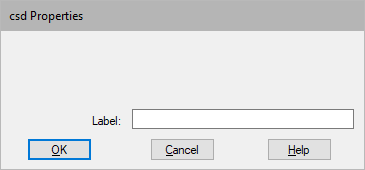## csd

Block Category: Matrix Operation

Description: The csd block (Cross Spectral Density) takes two time-series buffers and performs FFT on both. Then it performs a complex multiply of the first FFT by the complex conjugate of the seocnd FFT. The resulting magnitude and phase are put on the two block outputs.

The effect of multiplying by the complex conjugate is to give a zero imaginary value when the two complex points are equivalent. This means that if both signals share a similar frequency magnitude peak and are in phase, the resulting phase of the multiply will be 0. If there is a phase difference, it will be seen in the phase output.Label: Indicates a user-defined block label that appears when View > Block Labels is activated.